# How much heat energy is required to convert 49.7 g of solid ethanol at –114.5 °C...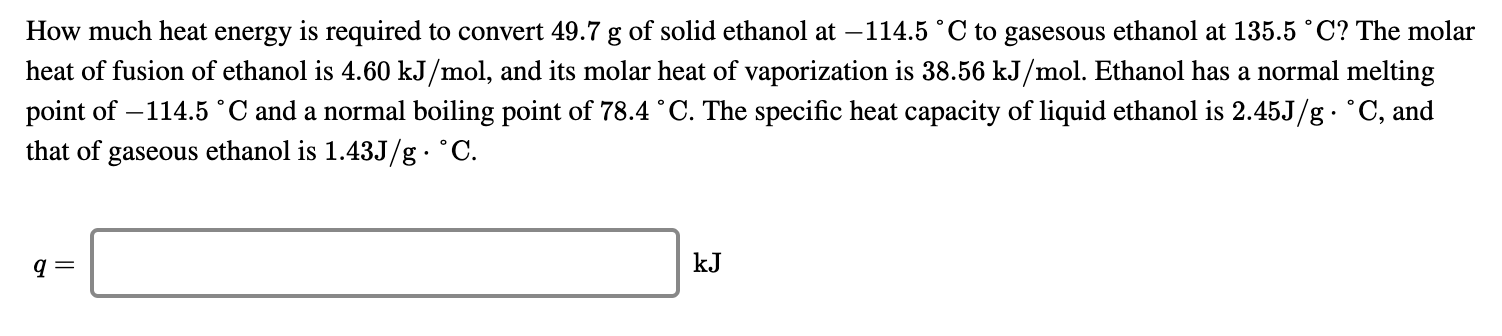How much heat energy is required to convert 49.7 g of solid ethanol at –114.5 °C to gasesous ethanol at 135.5 °C? The molar heat of fusion of ethanol is 4.60 kJ/mol, and its molar heat of vaporization is 38.56 kJ/mol. Ethanol has a normal melting point of -114.5 °C and a normal boiling point of 78.4 °C. The specific heat capacity of liquid ethanol is 2.45J/g. °C, and that of gaseous ethanol is 1.43J/g • °C. kᎫ

Ti = -114.5 oC
Tf = 135.5 oC
here
Hfus = 4.6KJ/mol =
4600J/mol

Lets convert mass to mol
Molar mass of C2H5OH = 46.068 g/mol
number of mol
n= mass/molar mass
= 49.7/46.068
= 1.0788 mol

Heat required to convert solid to liquid at -114.5 oC
Q1 = n*Hfus
= 1.0788 mol *4600 J/mol
= 4962.6639 J

Cl = 2.45 J/g.oC

Heat required to convert liquid from -114.5 oC to 78.4 oC
Q2 = m*Cl*(Tf-Ti)
= 49.7 g * 2.45 J/g.oC *(78.4--114.5) oC
= 23488.4685 J

Hvap = 38.56KJ/mol =
38560J/mol

Heat required to convert liquid to gas at 78.4 oC
Q3 = n*Hvap
= 1.0788 mol *38560 J/mol
= 41600.0695 J

Cg = 1.43 J/g.oC

Heat required to convert vapour from 78.4 oC to 135.5 oC
Q4 = m*Cg*(Tf-Ti)
= 49.7 g * 1.43 J/g.oC *(135.5-78.4) oC
= 4058.1541 J

Total heat required = Q1 + Q2 + Q3 + Q4
= 4962.6639 J + 23488.4685 J + 41600.0695 J + 4058.1541 J
= 74109 J
= 74.11 KJ

#### Earn Coin

Coins can be redeemed for fabulous gifts.

Similar Homework Help Questions
• ### How much heat energy is required to convert 15.0 g of solid ethanol at -114.5 °C...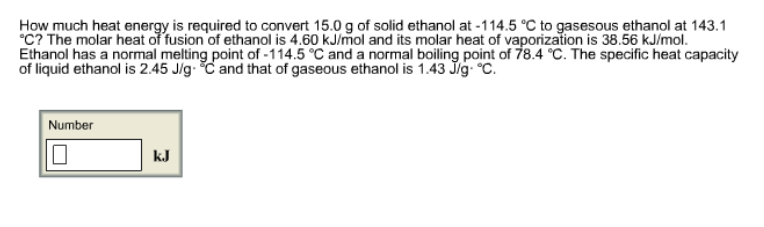How much heat energy is required to convert 15.0 g of solid ethanol at -114.5 °C to gasesous ethanol at 143.1 °C? The molar heat of fusion of ethanol is 4.60 kJ/mol and its molar heat of vaporization is 38.56 kJ/mol. Ethanol has a normal melting point of -114.5 °C and a normal boiling point of 78.4 °C. The specific heat capacity of liquid ethanol is 2.45 J/g·°C and that of gaseous ethanol is 1.43 J/g·°C. How much heat energy...

• ### How much heat energy is required to convert 19.8 g of solid ethanol at –114.5 °C...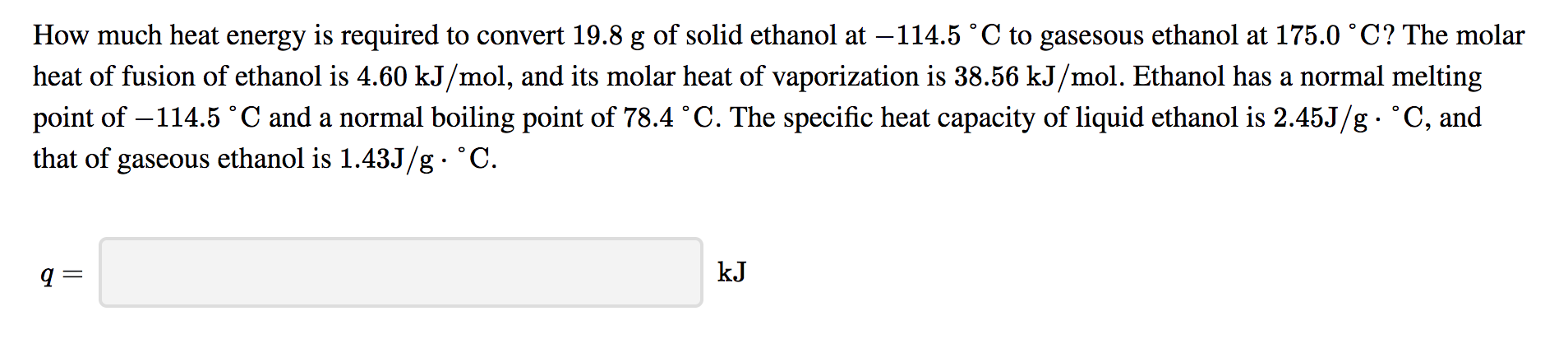How much heat energy is required to convert 19.8 g of solid ethanol at –114.5 °C to gasesous ethanol at 175.0 °C? The molar heat of fusion of ethanol is 4.60 kJ/mol, and its molar heat of vaporization is 38.56 kJ/mol. Ethanol has a normal melting point of –114.5 °C and a normal boiling point of 78.4 °C. The specific heat capacity of liquid ethanol is 2.45J/g • °C, and that of gaseous ethanol is 1.43J/g. °C.

• ### Resources Hint Check Answer Question 8 of 10 > How much heat energy is required to...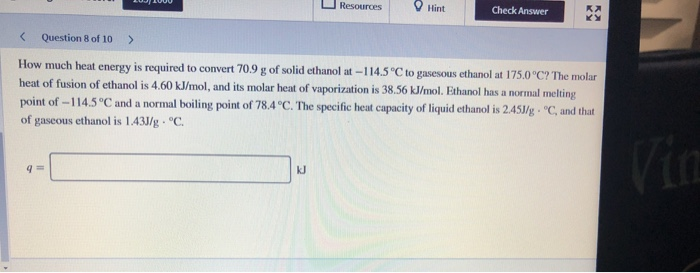Resources Hint Check Answer Question 8 of 10 > How much heat energy is required to convert 70.9 g of solid ethanol at-114.5 °C to gasesous ethanol at 175.0°C? The molar heat of fusion of ethanol is 4.60 kJ/mol, and its molar heat of vaporization is 38.56 kJ/mol. Ethanol has a normal melting point of -114.5°C and a normal boiling point of 78.4°C. The specific heat capacity of liquid ethanol is 2.453/g • °C, and that of gaseous ethanol is...

• ### What amount of thermal energy (in kJ) is required to convert 44.1 g of ethanol at...

What amount of thermal energy (in kJ) is required to convert 44.1 g of ethanol at -178 °C completely to gaseous ethanol at 119 °C? The melting point of ethanol is -114 °C and its normal boiling point is 78 °C. The heat of fusion of ethanol is 5.0 kJ mol-1 The heat of vaporization of ethanol at its normal boiling point is 35.0 kJ mol-1 The specific heat capacity of solid ethanol is 1.05 J g-1 °C-1 The specific...

• ### How much heat energy is required to convert 45.2 g of a solid iron at 28...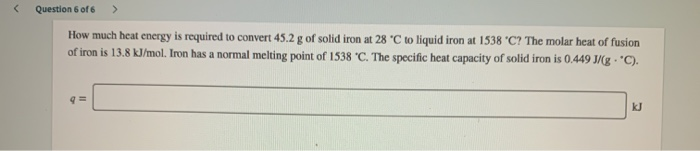How much heat energy is required to convert 45.2 g of a solid iron at 28 C to liquid iron at 1538 C? The molar heat of fusion of iron is 13.8 kJ/mol. Iron has a normal melting point of 1538 C. The specific heat capacity of solid iron is 0.449 J/ (g • C). Question 6 of 6 > How much heat energy is required to convert 45.2 g of solid iron at 28 °C to liquid iron at...

• ### How much heat energy is required to convert 57.6 g of solid iron at 21 °C...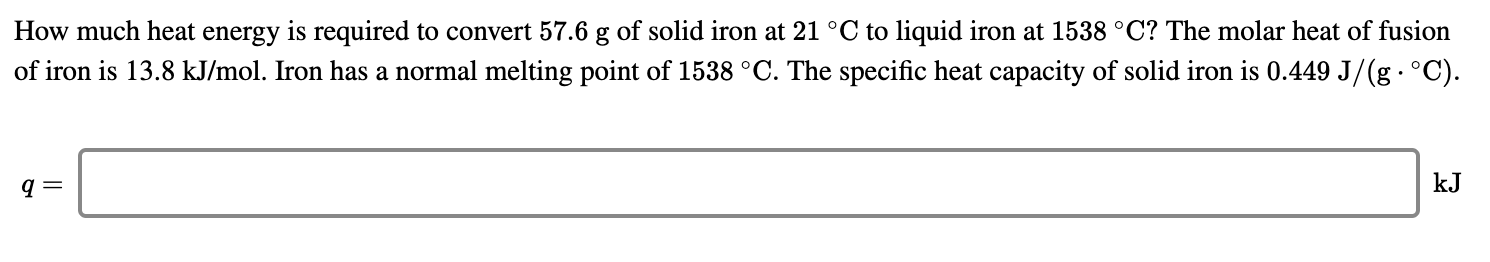How much heat energy is required to convert 57.6 g of solid iron at 21 °C to liquid iron at 1538 °C? The molar heat of fusion of iron is 13.8 kJ/mol. Iron has a normal melting point of 1538 °C. The specific heat capacity of solid iron is 0.449 J/(g· °C). 9 = kJ

• ### How much heat energy is required to convert 51.4 g of solid iron at 28 °C...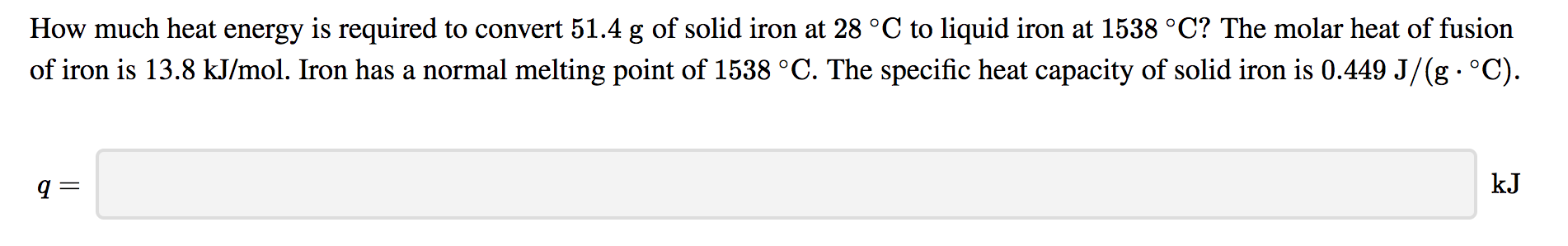How much heat energy is required to convert 51.4 g of solid iron at 28 °C to liquid iron at 1538 °C? The molar heat of fusion of iron is 13.8 kJ/mol. Iron has a normal melting point of 1538 °C. The specific heat capacity of solid iron is 0.449 J/(g °C) kJ

• ### How much heat energy is required to convert 94.5 g of solid iron at 26 °C...

How much heat energy is required to convert 94.5 g of solid iron at 26 °C to liquid iron at 1538 °C? The molar heat of fusion of iron is 13.8 kJ/mol. Iron has a normal melting point of 1538 °C. The specific heat capacity of solid iron is 0.449 J/g·°C?

• ### Determine the amount of heat required to convert 500.0 g of liquid ethanol at 25.0°C into...

Determine the amount of heat required to convert 500.0 g of liquid ethanol at 25.0°C into gaseous ethanol at 125°C. Use the following information on ethanol (C2H5OH) to calculate the amount of heat for each of the steps. Melting Point = −117°C Boiling Point = 78.3°C Molar Heat Capacities: Csolid =113 J/mol °C Cliquid = 420 J/mol °C ΔHfusion = 5.02 kJ/molΔHvaporization = 39.3 kJ/mol

• ### Calculate the total quantity of heat required to convert 25.0 g of liquid CCl 4 (...

Calculate the total quantity of heat required to convert 25.0 g of liquid CCl 4 ( l ) from 35.0°C to gaseous CCl 4 at 76.8°C (the normal boiling point for CCl 4 ). The specific heat of CCl 4 ( l ) is 0.857 J/(g · °C), its heat of fusion is 3.27 kJ/mol, and its heat of vaporization is 29.82 kJ/mol.

Free Homework App